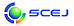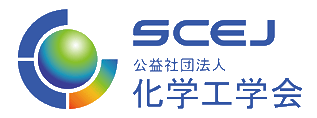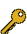\$B2=3X9)3X2qBh(B87\$BG/2q(B 2022.3.16(\$B?e(B) - 3.18(\$B6b(B) \$B?@8MBg3X(B \$BDa9CBh(B1\$B%-%c%s%Q%9(B / \$B%*%s%i%\$%s(B English page
Last Update: 2023-09-22 01:47:28

## 7-e. \$B%a%G%#%+%k(B

\$B:G=*99?7F|;~!'(B2022-06-14 16:59:01\$B\$3\$NJ,N`\$G\$h\$/;H\$o\$l(B
\$B\$F\$\$\$k%-!<%o!<%I(B
\$B%-!<%o!<%I(B\$B
Image analysis3\$B7o(BLaboratory automation3\$B7o(BCancer cells2\$B7o(BBiomaterial2\$B7o(Bhydrogel2\$B7o(Bmesenchymal stem cell2\$B7o(Bdrug delivery1\$B7o(B\$B\$BHV9f(B\$B9V1iBjL\!?H/I=\$B%-!<%o!<%I(B\$BH/I=7A<0(B
8\$B\$,\$s:YK&FbIt\$G<+8JAH?%2=\$7%"%]%H!<%7%9;`\$r0z\$-5/\$3\$9%Z%W%A%I;i
(Kobe U.) \$B!{(B(\$B@5(B)\$B?9ED(B \$B7rB@(B\$B!&(B \$B@>B<(B \$B9a2;(B\$B!&(B (\$B3X(B)\$B;3K\(B \$BfFB@(B\$B!&(B (\$B3X(B)\$B@6?e(B \$B\$J\$D\$_(B\$B!&(B \$B@D0f(B \$B5.G7(B\$B!&(B \$BEDB<(B \$B8|IW(B\$B!&(B (\$B@5(B)\$B4];3(B \$BC#@8(B
peptide lipid
self-assembly
cancer
P
17\$B%A%m%7%s4^M-%Z%W%A%I;i
(\$B?@8MBg1!9)(B) \$B!{(B(\$B3X(B)\$B@6?e(B \$B\$J\$D\$_(B\$B!&(B (\$B3X(B)\$B6b8w(B \$B:L@c(B\$B!&(B \$BH,Be(B \$BJ~;R(B\$B!&(B (\$B@5(B)\$B?9ED(B \$B7rB@(B\$B!&(B (\$B@5(B)\$B4];3(B \$BC#@8(B
cancer cells
peptides
tyrosine kinase
P
76\$B7PHiKc?lLt\$K\$*\$1\$k?<6&>=8=>]\$NMxMQ(B
(\$BF1;V(\$B@5(B)\$BED86(B \$B5AO/(B\$B!&(B \$BCf@n(B \$BM:B@(B\$B!&(B (\$B@5(B)\$B>>K\(B \$BF;L@(B
Deep eutectic mixture
Topical anesthesia
Transdermal delivery
O
209Development of a Novel Chitosan-based Hydrogel as a Tissue Adhesive
(\$BElBg1!9)(B) \$B!{(B(\$B3X(B)\$Bhq(B \$B7J>g(B\$B!&(B (\$B3X(B)Athira Sreedevi Madhavikutty\$B!&(B (\$BElBg1!0e(B) (\$B@5(B)Arvind Kumar Singh Chandel\$B!&(B (\$BElBg1!0e(B/\$BElBg1!9)(B) (\$B@5(B)\$B0KF#(B \$BBgCN(B
chitosan hydrogel
P
254\$BFs=E4IO"7k%G%P%\$%9\$rMQ\$\$\$?7l4ILOJo%3%i!<%2%s%^%\$%/%m%A%e!<%V\$N:n@=(B
(\$B@iMUBg1!9)(B) \$B!{(B(\$B3X(B)\$BLb;3(B \$B4SB@(B\$B!&(B (\$B3X(B)\$B9bLZ(B \$B??0T(B\$B!&(B \$BL*ED(B \$BBg2m(B\$B!&(B (\$B@5(B)\$B;3ED(B \$B??@!(B\$B!&(B \$B1-F,(B \$BM}7C(B\$B!&(B (\$B@5(B)\$B4X(B \$B
Collagen
Vascular tissue
Microfluidic device
P
296\$B?@7P:YK&(B1\$B:YK&5!G=2r@O\$N\$?\$a\$NH/2P%Q%?!<%sDjNL2r@O(B
(\$BL>Bg1!AOLt(B) \$B!{(B(\$B3X(B)\$B5WED(B \$BBs3\$(B\$B!&(B (\$BEl5~;|7C2q0eBg(B) \$B?9Eg(B \$BH~3(;R(B\$B!&(B (\$BL>Bg1!AOLt(B) (\$B@5(B)\$B3*9>(B \$B7E(B\$B!&(B (\$BL>Bg1!AOLt(B/\$BL>Bg%J%N%i%\$%U%7%9%F%`8&(B) (\$B@5(B)\$B2CF#(B \$BN5;J(B
Neuron
Firing pattern
Single cell analysis
P
298\$B6a@V308w\$rMQ\$\$\$?\$,\$s%9%U%'%m%\$%I%b%G%kI>2A(B
(\$BL>Bg1!AOLt(B) \$B!{(B(\$B3X(B)\$BNS(B \$B:i4u(B\$B!&(B \$B1J0f(B \$BH~4u(B\$B!&(B (\$B@5(B)\$B3*9>(B \$B7E(B\$B!&(B (\$B=;M'EE9)(B) \$B8^==Mr(B \$BM[;R(B\$B!&(B \$B?{>B(B \$B42(B\$B!&(B (\$BL>Bg1!AOLt(B/\$BL>Bg%J%N%i%\$%U%7%9%F%`8&(B) (\$B@5(B)\$B2CF#(B \$BN5;J(B
Cancer spheroid
Near-infrared light
Image analysis
P
299\$BL~CeKI;_:`NA@_7W\$N\$?\$a\$N:YK&FC0[E*@\Ce8=>]I>2A%W%i%C%H%U%)!<%`\$N9=C[(B
(\$BL>Bg1!AOLt(B) \$B!{(B(\$B3X(B)\$BAz8EED(B \$B0lM%(B\$B!&(B (\$B3X(B)\$B?y;3(B \$B0!LpEM(B\$B!&(B (\$B@5(B)\$B3*9>(B \$B7E(B\$B!&(B (\$BL>Bg1!9)(B) \$B86(B \$B8w@8(B\$B!&(B (NIMS) \$B1'ET(B \$B9C0lO:(B\$B!&(B \$B1A86(B \$B=<9((B\$B!&(B (\$BL>Bg1!0e(B) \$B=oJ}(B \$BMu2N(B\$B!&(B \$B@.ED(B \$BM5;J(B\$B!&(B (\$BL>Bg1!AOLt(B/\$BL>Bg%J%N%i%\$%U%7%9%F%`8&(B) (\$B@5(B)\$B2CF#(B \$BN5;J(B
Biomaterial
Laboratory automation
Image analysis
P
30196\$B%&%'%k%W%l!<%H\$K\$*\$1\$k:YK&6Q0lGEr7o\$N:GE,2=(B
(\$BL>Bg1!AOLt(B) \$B!{(B(\$B3X(B)\$B:d(B \$B>-@.(B\$B!&(B \$B:#0f(B \$BM4B@(B\$B!&(B \$B1|B<(B \$BM4EM(B\$B!&(B (\$B@5(B)\$B3*9>(B \$B7E(B\$B!&(B (\$BL>Bg1!AOLt(B/\$BL>Bg%J%N%i%\$%U%7%9%F%`8&(B) (\$B@5(B)\$B2CF#(B \$BN5;J(B
Laboratory automation
Cell seeding
Image analysis
P
304\$B
(\$BL>Bg1!AOLt(B) \$B!{(B(\$B3X(B)\$B?y;3(B \$B0!LpEM(B\$B!&(B (\$B@5(B)\$B3*9>(B \$B7E(B\$B!&(B (\$B3X(B)\$BAz8EED(B \$B0lM%(B\$B!&(B (NIMS) \$B1'ET(B \$B9C0lO:(B\$B!&(B (\$BL>Bg1!0e(B) \$B=oJ}(B \$BMu2N(B\$B!&(B (NIMS) \$B1A86(B \$B=<9((B\$B!&(B (\$BL>Bg1!0e(B) \$B@.ED(B \$BM5;J(B\$B!&(B (\$BL>Bg1!AOLt(B/\$BL>Bg%J%N%i%\$%U%7%9%F%`8&(B) (\$B@5(B)\$B2CF#(B \$BN5;J(B
Biomaterial
High content analysis
Laboratory automation
P
325\$BJ"KlGE
(\$BElBg1!9)(B) \$B!{(B(\$B3X(B)\$B>>ED(B \$B97Bg(B\$B!&(B (\$B@5(B)\$BB@ED(B \$B@?0l(B\$B!&(B (\$BElBg1!0e(B/\$BElBg1!9)(B) (\$B@5(B)\$B0KF#(B \$BBgCN(B
drug delivery
mathematical model
peritoneal dissemination
P
329\$B%b%A!<%UO"7k7??M9)
(\$B0eLt7r1I8&(B) \$B!{(B(\$B@5(B)\$B2O86(B \$B@59@(B\$B!&(B \$BCfEh(B \$B63;R(B
chimeric receptor
tyrosine motif
signal transduction
O
348Development and Functional Evaluation of a Miniature Liver Model
(Kyushu U.) \$B!{(B(\$B3X(B)Uehara Uyeda Mario Kokichi\$B!&(B Wu Fanqi\$B!&(B Fukuda Yukako\$B!&(B (\$B@5(B)Sakai Yusuke\$B!&(B Shirakigawa Nana\$B!&(B (Kumamoto U.) Miyata Tatsunori\$B!&(B Nakao Yosuke\$B!&(B Yamao Takanobu\$B!&(B (Saga U.) Aishima Shinichi\$B!&(B (Kumamoto U.) Yamashita Yo-ichi\$B!&(B Baba Hideo\$B!&(B (Kyushu U.) (\$B@5(B)Ijima Hiroyuki
Decellularized Liver
Primary Human Hepatocyte
Cell Distribution
P
352\$B7PHi%^%i%j%"%o%/%A%s\$N3+H/\$*\$h\$S7PHiEjM?\$K\$h\$kLH1V1~Ez2r@O(B
(\$B6eBg1!9)(B) \$B!{(B(\$B3X(B)\$BEDCf(B \$B7IM\$(B\$B!&(B (\$B@5(B)\$BFnH*(B \$B9'2p(B\$B!&(B (\$B@5(B)\$B\$B!&(B (\$B@5(B)\$B?@C+(B \$BE5Jf(B\$B!&(B (\$B@5(B)\$B8eF#(B \$B2m9((B
Transdermal vaccine delivery
Malaria
Immunology
P
375\$B:YK&7ABV>pJs\$rMQ\$\$\$?G]CO8r49%G%6%\$%s%9%Z!<%9\$NM}2r(B
(\$BL>Bg1!AOLt(B) \$B!{(B(\$B3X(B)\$B?\$B!&(B \$BC]K\(B \$BM*?M(B\$B!&(B \$B0KF#(B \$BM':H(B\$B!&(B (\$B3X(B)\$B5WED(B \$BBs3\$(B\$B!&(B (\$B@5(B)\$B3*9>(B \$B7E(B\$B!&(B (\$BL>Bg1!AOLt(B/\$BL>Bg%J%N%i%\$%U%7%9%F%`8&(B) (\$B@5(B)\$B2CF#(B \$BN5;J(B
Mesenchymal Stem Cell
Quality by Design
Medium change
P
396\$B9ZAGH?1~\$rMxMQ\$7\$?9bJ,;R!&D6J,;R%O%\$%V%j%C%I%2%k\$NAO@=\$H5!G=2=(B
(\$B6eBg1!9)(B) \$B!{(B(\$B3X(B)\$BHu8}(B \$B0!LiEM(B\$B!&(B (\$B@5(B)\$B\$B!&(B (\$B@5(B)\$B8eF#(B \$B2m9((B\$B!&(B (\$B@5(B)\$B?@C+(B \$BE5Jf(B
hybrid-gel
enzymatic-reaction
functionalization
P
402Balance of anti-peritoneal adhesion, hemostasis, and operability of compressed bi-layer ultrapure alginate sponges
(U. Tokyo) \$B!{(B(\$B3X(B)Dai Yizhou\$B!&(B (\$B@5(B)Kumar Singh Chandel Arvind\$B!&(B Yoshida Hiromi\$B!&(B Nakamichi Akiko\$B!&(B Taniguchi Machiko\$B!&(B (\$B@5(B)Ohta Seiichi\$B!&(B (U. Tokyo/U. Tokyo Hospital) (\$B@5(B)Ito Taichi
Hemostat
Sponge
P
424\$B9ZAG2M66@-%"%k%V%_%s%2%k2=:^\$N3+H/\$H:YK&Jq3g\$X\$N1~MQ(B
(\$B:4@\$J]9b@l(B) \$B!{(B(\$B@5(B)\$B?9;3(B \$B9,M4(B\$B!&(B (\$B3X(B)\$B0f85(B \$BG53((B\$B!&(B (\$BD92,5;2JBg(B) \$BFsIS(B \$B>-D*(B\$B!&(B \$B:4F#(B \$BH~6k(B\$B!&(B \$B:4F#(B \$BIp;K(B\$B!&(B (\$B6eBg1!9)(B) (\$B@5(B)\$B8eF#(B \$B2m9((B\$B!&(B (\$B@5(B)\$B?@C+(B \$BE5Jf(B
hydrogel
albumin
enzyme
P
514\$B;ih8z2L(B
(\$B6eBg1!9)(B) Pugoh Santoso\$B!&(B \$B!{(B(\$B@5(B)\$BFnH*(B \$B9'2p(B\$B!&(B (\$BN0BgG@(B) \$B@PNf(B \$BM*8g(B\$B!&(B (\$B6eBg1!9)(B) (\$B3X(B)\$BC+8}(B \$B9@@?(B\$B!&(B (\$B3X(B)\$B:4F#(B \$BVE(B\$B!&(B (\$B@5(B)\$B8eF#(B \$B2m9((B\$B!&(B (\$BN0BgG@(B) 髙\$BEg(B \$BCRLi(B\$B!&(B \$BJ?NI(B \$BEl5*(B\$B!&(B (\$B6eBg1!9)(B) (\$B@5(B)\$B?@C+(B \$BE5Jf(B
Chitinase
Lipidation
Antifungal activity
O
560\$B%U%#%k%`4pHW(BC2C12\$B6ZAH?%\$rMQ\$\$\$?%+%j%&%`\$N1F6A(B
(\$BBg9)Bg@8L?9)(B) \$B!{(B(\$B3X(B)\$B4]LS(B \$BM[B@(B\$B!&(B (\$BBg9)Bg1!9)@8L?9)(B) (\$B3X(B)\$B2O>e(B \$BMZF`(B\$B!&(B \$B2E1Y(B \$BM&B@(B\$B!&(B (\$B@5(B)\$BD9?9(B \$B1QFs(B\$B!&(B (\$B@5(B)\$B:j;3(B \$BN<0l(B
C2C12
Muscle atrophy
potassium
P
579\$B7V8wLH1V%;%s%5!<(BCoiled Quenchbody\$B\$N\$,\$s8w@~NO3XNEK!\$X\$N1~MQ(B
(Tokyo Tech) \$B!{(B(\$B3X(B)\$BDX(B \$Bt%2L(B\$B!&(B (\$B3X(B)\$B0BED(B \$B5.?.(B\$B!&(B (\$B@5(B)\$B\$B!&(B \$BKL8}(B \$BE/Li(B\$B!&(B (\$B@5(B)\$B>eED(B \$B9((B
antibody
Coiled Quenchbody
Photodynamic Therapy
P
609\$B%*!<%H%/%l!<%VLG6]\$K\$h\$jD4@=\$5\$l\$k%-%H%5%s%2%k\$N3+H/(B
(\$B(\$B3X(B·\$B5;4p(B)\$B;32<(B \$BM4E5(B\$B!&(B (\$B@5(B)\$BBg3Q(B \$B5A9@(B\$B!&(B (\$B@5(B)\$B5HED(B \$B>;90(B\$B!&(B (\$B@5(B)\$BIp0f(B \$B9'9T(B
chitosan
hydrogel
autoclaving
P
612\$B@8BNE,9g@-%\$%*%s1UBN1U>=%-%c%j%"\$rMQ\$\$\$?7PHi5[<}@=:^\$N3+H/(B
(\$B6eBg1!9)(B) \$B!{(B(\$B3X(B)\$B86(B \$B9>4u(B\$B!&(B (\$B@5(B)\$B?@C+(B \$BE5Jf(B\$B!&(B (\$B@5(B)\$B8eF#(B \$B2m9((B
Drug Delivery System
Ionic Liquid
Liquid Crystal
P
613\$B;@AGG;EY\$N0c\$\$\$,G]M\9|3J6Z:YK&\$KM?\$(\$k1F6A(B
(\$BL>Bg1!9)(B) \$B!{(B(\$B3X(B)\$BM-K\(B \$B2CF`3((B\$B!&(B (\$B@5(B)\$B=);3(B \$BM5OB(B\$B!&(B (\$B@5(B)\$BK\B?(B \$BM5G7(B\$B!&(B (\$B@5(B)\$B@6?e(B \$B0l7{(B
Skeletal muscle cells
Oxygen concentration
Differentiation
P
633\$B46@w>IM=KI\$rL\E*\$H\$7\$?Cm
(\$B6eBg1!9)(B) \$B!{(B(\$B3X(B)\$B8EED(B \$B??M}(B\$B!&(B (\$B3X(B)\$B>.:d(B \$B=(EM(B\$B!&(B (\$B@5(B)\$B8eF#(B \$B2m9((B
transdermal
vaccine
patch
P
647miRNA\$B%Q%M%k\$rMQ\$\$\$?(BCAR-T\$B:YK&NEK!\$N%3%s%Q%K%*%s?GCG%b%G%k\$N3+H/(B
(\$BElBg9)(B) \$B!{(B(\$B3X(B)濵\$BED(B \$BM|=m(B\$B!&(B (\$BElBg1!9)(B) (\$B@5(B)\$BCfB<(B \$BG5M};R(B\$B!&(B (\$B0&CN\$,\$s%;(B) \$BdFC+(B \$BM&5*(B\$B!&(B (\$BL@<#BgM}9)(B) (\$B@5(B)\$B6b;R(B \$B90>;(B\$B!&(B (\$BElBg1!9)(B/JST\$B\$5\$-\$,\$1(B) (\$B@5(B)\$BB@ED(B \$B@?0l(B
companion diagnostics
miRNA
CAR-T cell therapy
P
657\$B:YK&@\Ce%Z%W%A%I=\$>~B?9&
(\$BElBg1!9)(B) \$B!{(B(\$B3X(B)\$BLn4V(B \$B1_(B\$B!&(B (\$B3X(B)\$BBgLZ(B \$BM*0lO/(B\$B!&(B (\$B002=@.(B) (\$BK!(B)\$B86G<(B \$B90Bg(B\$B!&(B (\$BK!(B)\$BB<2,(B \$B8,(B\$B!&(B (\$BK!(B)\$B86(B \$BM:0l(B\$B!&(B (\$BElBg1!9)(B) (\$B@5(B)\$B0KF#(B \$BBgCN(B
mesenchymal stem cell
microcarrier
RGD
P
669\$B\$,\$s:YK&M7AvAK32\$K8~\$1\$?(BEWI-2\$BM3Mh%F%H%i%9%Q%K%s(BCD81\$B7k9g@-%Z%W%A%I\$NC5:w(B
(\$BEl9)Bg(B) \$B!{(B(\$B3X(B)\$BGv>l(B \$B7=(B\$B!&(B \$B%9%o%C%?%J!<%i%C%H(B \$B%?%J%o%C%H(B\$B!&(B (\$B@5(B)\$BEDCf(B \$BM47=(B\$B!&(B (\$B@5(B)\$BBg2OFb(B \$BH~F`(B
CD81 protein
Peptide
Cancer cells
P
699\$B%^%\$%/%m%W%i%9%A%C%/\$N?MBN1F6AI>2A(B
(\$BElBg1!9)(B) \$B!{(B(\$B3X(B)\$BNkLZ(B \$BM%@5(B\$B!&(B (\$B@5(B)\$B0pB<(B \$B91N<(B\$B!&(B (\$B3\$(B)\$B%@%N%"(B \$B%^%7%e!<(B\$B!&(B (\$B@5(B)\$B@>@n(B \$B>;51(B\$B!&(B (\$B@5(B)\$B
microplastic
in vitro
toxicity
P

\$B9V1i?=\$79~\$_0lMw(B(\$B%7%s%]%8%&%`HV9f!&9V1iJ,N`HV9fJL(B)

\$B

This page was generated byeasp 2.47; update.pl 2.37 (C)1999-2015 kawase